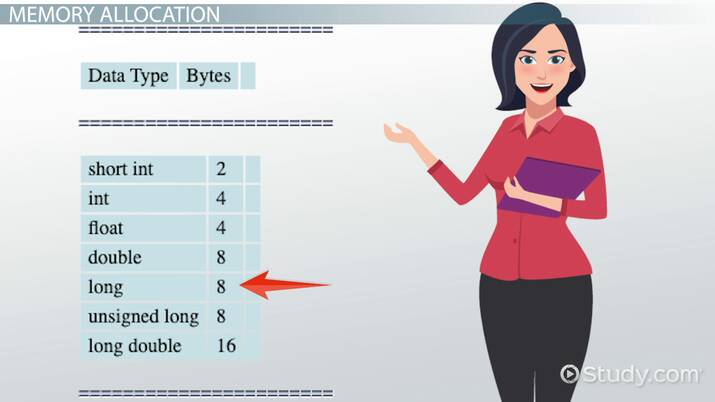Computer Science Courses / Course / Chapter

# Numeric Data Types in C++ Programming

Lesson Transcript
Instructor: Edward Lavieri

Ed has created and taught college courses since 2002 and has a Doctorate of Computer Science and three MS degrees. He has authored several tech books.

In this lesson, we will explore the numeric data types in the C++ programming language. Ranges of each numeric data type will be reviewed as well as their required memory allocation. Updated: 02/04/2023

## C++ Numeric Data Types

C++ is a core programming language that makes great use of numeric data types. These numeric data types can be categorized as integers (whole numbers) and floating points (which have decimal points). Let's look at each category of numeric data types.An error occurred trying to load this video.

Try refreshing the page, or contact customer support.

Coming up next: Arithmetic Operations in C++ Programming

### You're on a roll. Keep up the good work!

Replay
Your next lesson will play in 10 seconds
• 0:04 C++ Numeric Data Types
• 0:21 Integers
• 1:54 Floating Points
• 2:24 Memory Allocation
• 2:55 Lesson Summary
Save Timeline
Autoplay
Autoplay
Speed Speed

## Integers

Integers, referred to in code as int, come in several forms. A short int can be any number in the range of -32,768 to 32,767. A regular int, or just int, has a much larger range of -2,147,483,648 to 2,147,483,647.

A long int, referred to as long or double, has a range of -9,223,372,036,854,775,808 to 9,223,372,036,854,775,807.

It's important to realize that there is no single standard for numeric data types in C++ and the names and range values can be slightly different depending on the implementation and processor. What is provided in this lesson are the prevailing ranges.

We can execute the following lines of code to determine the range of each data type.

`cout << 'short int Min/Max : ' << SHRT_MIN << ' / ' << SHRT_MAX << endl;cout << 'int Min/Max : ' << INT_MIN << ' / ' << INT_MAX << endl;cout << 'long Min/Max : ' << LONG_MIN << ' / ' << LONG_MAX << endl;`

The output is as follows:

`short int Min/Max : -32768 / 32767int Min/Max : -2147483648 / 2147483647long Min/Max : -9223372036854775808 / 9223372036854775807`

Integers can also be signed (support for negative numbers) or unsigned. We can use the following code to determine the maximum value of unsigned integers:

`cout << 'unsigned short int Max : ' << USHRT_MAX << endl;cout << 'unsigned int Max : ' << UINT_MAX << endl;cout << 'unsigned long Max : '<< ULONG_MAX << endl;`

The output of this code is as follows:

`unsigned short int Max : 65535unsigned int Max : 4294967295unsigned long Max : 18446744073709551615`

## Floating Points

Floating points can have a decimal, which provide a greater level of precision over integers.

There are three types of floating point numbers in C++: float (the smallest), double (twice the size of floats), and long double (twice the size of doubles). The following code will generate output to display the minimum and maximum value of the float data types.

`cout << '\tFloat \t\t' << 'Double \t\t' << 'Long Double' << endl;cout << 'Min : ' << FLT_MIN << '\t' << DBL_MIN << '\t' << LDBL_MIN << endl;cout << 'Max : ' << FLT_MAX << '\t' << DBL_MAX << '\t' << LDBL_MAX << endl;`

The output for the preceding code is provided here:

`Float Double Long DoubleMin : 1.17549e-38 2.22507e-308 3.3621e-4932Max : 3.40282e+38 1.79769e+308 1.18973e+4932`

## Memory Allocation

Numeric data types, like other data types, require memory allocation whenever a variable is defined. How much memory is allocated depends on the data type. A good programming practice is to use the smallest data type possible for any given situation to conserve memory.

To unlock this lesson you must be a Study.com Member.

### Register to view this lesson

Are you a student or a teacher?

Back

### Resources created by teachers for teachers

Over 30,000 video lessons & teaching resources‐all in one place.Video lessonsQuizzes & WorksheetsClassroom IntegrationLesson Plans

I would definitely recommend Study.com to my colleagues. It’s like a teacher waved a magic wand and did the work for me. I feel like it’s a lifeline.

Jennifer B.
TeacherCreate an account to start this course today
Used by over 30 million students worldwide